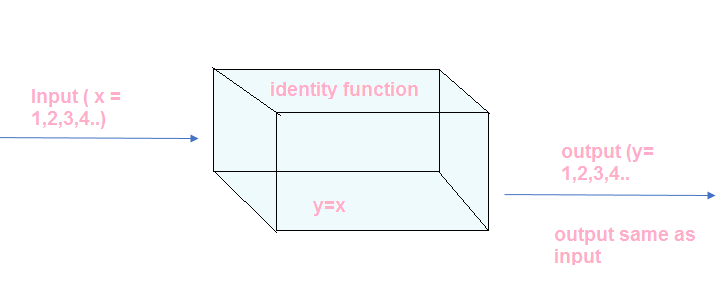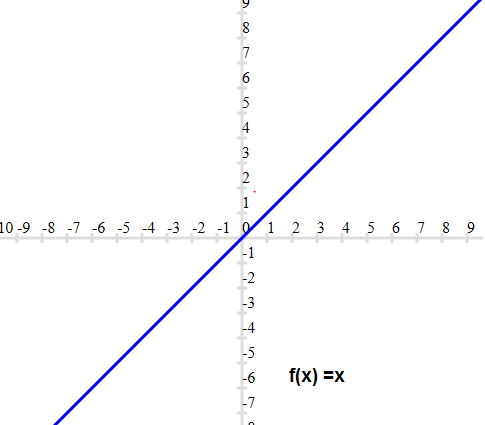# Identity Function

## Standard Real Function and Their graphs

We will be exploring some Standard Real Function and Their graphs in next few pages like
a. Identity function
b. Constant function
c. Linear function
e. cubic function
f. polynomial function
g. rational function
h. Modulus function

We will discussing their domain ,range, graph and properties in details.
Let's get started with first function

## Identity Function

We have already seen in previous grades that Identity of addition ,subtraction ,multiplication are number which when operated on the elements does not change the value of the element.
Example
$1 + 0 =1$
$2 + 0 =2$
Multiplicatve identity
$5 \times 1 =5$

Now Identity function are similar type of thing.
Identity Function is defined as the real valued function $f : R \rightarrow R$ , y = f(x) = x for each $x \in R$
So ,this function basically associate each real number to itself### Domain and Range of the Identity Function

For $f : R \rightarrow R$

Domain = R
Range = R
Co-domain and Range are equal set

### Graph of the Identity Function

We can draw the graph on the Cartesian plane with value of x on the x-axis and value of y=f(x) on the y-axis. We can plot the point and join the point to obtain the graph. Here in case of the identity function,the graph will be a straight line passing through the originThe straight line makes an angle $45^o$ with the x-axis

## Other Examples of Identity Functions

So far, we observe the identity function for the whole set of Real number. But Identity function can also be defined for the subset of the real numbers also

We denote these by capital letter I

Example -1
Let A = {1,2,3,4,5,6}
Then Identity function on set A will be defined as
$I_A : A \rightarrow A, I_A=x , x \in A$
for $x=1 ,I_A(1) =x=1$
for $x=2 ,I_A(1) =x=2$
for $x=3 ,I_A(1) =x=3$
for $x=4 ,I_A(1) =x=4$
for $x=5 ,I_A(1) =x=5$

Domain,Range and co-domain will be Set A

Example -2
Let A = R -{1/2}
Then Identity function on set A will be defined as
$I : A \rightarrow A, I_A=x , x \in A$

Domain,Range and codomain will be Set A

Example -3
A identity function can be defined as
$I : Z \rightarrow R, I_A=x , x \in Z$

In this case Domain,Range will be Z and co-domain will be R

### Quiz Time

Question 1.
Find the value of the function $f(x) = \frac {x-5}{x-3}$ at x=0
A.3/5
B. 0
C.5/3
D. 1
Question 2.
If f (x) = px + q, where p and q are integers, f (-1) = - 5 and f (3) = 3, then p and q are equal to ?
A. p=2,q=-3
B. p-=2,q=3
C. p=1,q=3
D. p=-2,q=-3
Question 3.
if $f(x) =\frac {1}{2x+1}$ ,then
A. $f[f(x] =\frac {2x-1}{2x+3}$
B. $f[f(x] =\frac {2x-1}{2x-3}$
C. $f[f(x] =\frac {2x+1}{2x-3}$
D. $f[f(x] =\frac {2x+1}{2x+3}$
Question 4.
Let $f(x) =2^x$,find the value of $\frac {f(2) -f(1)}{2 -1}$
A. 4
B. 2
C. 0
D. 1
Question 5.
if $f(x) =\frac {x+1}{x-1}$ ,then
A. $f[f(x] =\frac {x-1}{x+1}$
B. $f[f(x] =x$
C.$f[f(x] =-x$
D.$f[f(x] =\frac {1-x}{1+x}$
Question 6.
Find the Range and domain of the function $f(x) =x$
A. Domain = R, Range =R
B. Domain = R - {0}, Range = R
C. Domain = R - {0}, Range = {-1/2}
D. Domain = R - {1}, Range = R